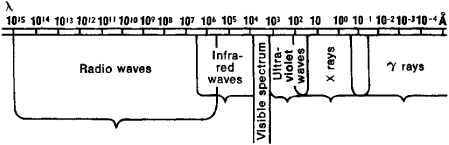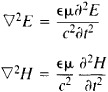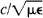# Electromagnetic Wave

Also found in: Dictionary, Thesaurus, Medical, Legal, Financial, Acronyms, Wikipedia.

## Electromagnetic wave

A disturbance, produced by the acceleration or oscillation of an electric charge, which has the characteristic time and spatial relations associated with progressive wave motion. A system of electric and magnetic fields moves outward from a region where electric charges are accelerated, such as an oscillating circuit or the target of an x-ray tube. The wide wavelength range over which such waves are observed is shown by the electromagnetic spectrum. The term electric wave, or hertzian wave, is often applied to electromagnetic waves in the radar and radio range. Electromagnetic waves may be confined in tubes, such as wave guides, or guided by transmission lines. They were predicted by J. C. Maxwell in 1864 and verified experimentally by H. Hertz in 1887. See Electromagnetic radiation

## Electromagnetic Wave

an electromagnetic oscillation that propagates in space at a finite speed.

The existence of electromagnetic waves was predicted by M. Faraday in 1832. In 1865, J. Maxwell showed theoretically that electromagnetic oscillations propagate in a vacuum at the speed of light c in all directions from a source rather than remaining localized in space. From the circumstances that the velocity of electromagnetic-wave propagation in a vacuum is equal to the speed of light, Maxwell concluded that light is electromagnetic waves. In 1888, Maxwell’s theory of electromagnetic waves was confirmed in experiments performed by H. Hertz. The experimental confirmation by Hertz played a decisive role in the acceptance of the theory.

Maxwell’s theory made possible a single approach to the description of radio waves, light, X rays, and gamma radiation. Such emissions were found to be electromagnetic waves with different wavelengths rather than radiations of different natures. The relation between the frequency ω of oscillations of an electric field E and magnetic field H and the wavelength λ of an electromagnetic wave is given by the equation λ = 2πc/ω. Radio waves, X rays, and gamma radiation are included in a single electromagnetic-wave spectrum (Figure 1). No sharp boundaries separate adjacent regions of the spectrum.Figure 1. The electromagnetic-wave spectrum

The characteristics of electromagnetic waves and the laws governing the generation and propagation of such waves are described by Maxwell’s equations. If electric charges e and currents I exist in some region of space, the variation of e and I with time t results in the radiation of electromagnetic waves. The velocity of electromagnetic-wave propagation is substantially affected by the medium in which the waves propagate. Electromagnetic waves may undergo refraction. Dispersion occurs in real media. Near inhomogeneities, diffraction is observed, as well as interference between incident and reflected waves, total internal reflection, and other phenomena that are characteristic of waves of any nature. The spatial distribution of electromagnetic fields, the time dependences E(t) and H(t), the type of polarization (seePOLARIZATION OF WAVES), and other characteristics of electromagnetic waves are specified by, on the one hand, the nature of the radiation source and, on the other hand, the properties of the medium in which the waves propagate. The time dependences E(t) and H(t) determine the type of wave, that is, whether a wave is plane or spherical.

In the case of a homogeneous and isotropic medium, far from the charges and currents that generate an electromagnetic field, Maxwell’s equations yield the wave equationswhere ∊ is the dielectric constant of the medium, μ is the permeability of the medium, and ∇2 is the Laplace operator. The equations describe the propagation of monochromatic plane electro-magnetic waves, for which

E = E0 cos(kr – ωt + φ)

H = H0 cos(kr – ωt + φ)

where E0 is the amplitude of the electric field oscillations, H0 is the amplitude of the magnetic field oscillations, ω is the frequency of the oscillations, φ is an arbitrary phase angle, k is a wave vector, and r is the radius vector of a point.

If a medium is anisotropic or contains surfaces on which its electrical or magnetic properties vary or if conductors are present in space, the type of electromagnetic waves that are generated and that propagate may be substantially different from linearly polarized plane waves. Electromagnetic waves may propagate along guiding surfaces (such waves are called surface waves), in transmission lines, or in cavities formed by walls that are good conductors (seeWAVE GUIDE, LIGHT GUIDE, and QUASI OPTICS).

The nature of the time variation of E and H is determined by the variation of the current I and the charges e that generate electromagnetic waves. However, in the general case, the shape of a wave does not conform to either I (t) or e (t). The shape of the waves exactly duplicates the shape of the current only in the case where the waves propagate in a linear medium, that is, in a medium whose electrical and magnetic properties are not functions of E and H. The simplest case of this type is the generation and propagation of electromagnetic waves in homogeneous and isotropic space by means of a Hertzian oscillator, which is a conductor of length I ≪ λ. through which a current I = I0 sin ωt flows. At a distance much greater than λ from the oscillator, a radiation zone is formed in which spherical electromagnetic waves propagate. Such waves are transverse waves and are linearly polarized. In the case of an anisotropic medium, the polarization may change (seeRADIO WAVES, RADIATION AND RECEPTION OF).

In isotropic space, the velocity v of harmonic electromagnetic waves, which is known as the phase velocity, is equal to. In the presence of dispersion, the rate c of energy transfer, or the group velocity, may differ from v. The flux density S of the energy transferred by electromagnetic waves is given by the Poynting vector S = (c/4π)[EH]. Since the vectors E and H and the wave vector form a right-handed system in an isotropic medium, the direction of S is the same as the direction of electromagnetic-wave propagation. In anisotropic media, including regions near conducting surfaces, the direction of S may not be the same as the direction of wave propagation.

The advent of quantum generators, especially lasers, made it possible to obtain, in electromagnetic waves, electric fields that are comparable to those within atoms. The attainment of such fields has resulted in the development of the nonlinear theory of electromagnetic waves. When an electromagnetic wave propagates in a nonlinear medium, that is, in a medium in which ∊ and μ are functions of E and H, the shape of the wave changes. If the dispersion is low, higher harmonics are generated as the electromagnetic wave propagates and the shape of the wave is gradually distorted. For example, a shock wave may be formed after a sinusoidal electromagnetic wave traverses a characteristic length, the value of which is determined by the medium’s degree of nonlinearity.

A shock wave is characterized by abrupt changes, or discontinuities, of E and H and the subsequent smooth return of E and H to their initial values. An electromagnetic shock wave propagates without substantial changes in its shape; abrupt changes are smoothed out mainly by damping. Most nonlinear media in which electromagnetic waves propagate without being strongly absorbed have a considerable dispersion, which prevents the formation of shock waves. Therefore, shock waves may be formed only in the wavelength region that ranges from a few centimeters to long waves. In the presence of dispersion, generated higher harmonics propagate at a different velocity and the shape of the original wave is not substantially distorted in a nonlinear medium. Intense harmonics may be generated and may interact with the original wave only when specially selected dispersion formulas are applicable (seeNONLINEAR OPTICS and OPTICAL PARAMETRIC OSCILLATOR).

Electromagnetic waves in different wavelength regions are characterized by different methods of generation and detection and interact with matter in different ways. The emission and absorption of electromagnetic waves ranging from the longest waves to infrared radiation are reasonably well described by the equations of electrodynamics. At higher frequencies, processes of a substantially quantum nature dominate. In the optical region and especially in the X-ray and gamma-ray regions, the emission and absorption of electromagnetic waves may be described only on the basis of concepts that take into account the discreteness of the processes considered.

Quantum field theory considerably augmented the concept of electromagnetic waves. In many cases, electromagnetic radiation behaves like a flux of quasiparticles rather than like an ensemble of electromagnetic waves with a frequency ω and a wave vector k. The quasiparticles are photons, which have an energy ℰ = ℏω and a momentum p = ℏω/c = ℏk, where ℏ is Planck’s constant. The wave properties of electromagnetic radiation are manifested in, for example, diffraction and interference; the corpuscular properties are manifested in photoelectric effects and in the Compton effect.

### REFERENCES

Tamm, I. E. Osnovy teorii elektrichestva, 9th ed. Moscow, 1976.
Landau, L. D., and E. M. Lifshits. Teoriia polia, 6th ed. Moscow, 1973. (Teoreticheskaia fizika, vol. 2.)
Landau, L. D., and E. M. Lifshits. Elektrodinamika sploshnykh sred. Moscow, 1959.
Landsberg, G. S. Optika, 5th ed. Moscow, 1976.

V. V. MIGULIN

## electromagnetic wave

[i¦lek·trō·mag′ned·ik ′wāv]
(electromagnetism)
A disturbance which propagates outward from any electric charge which oscillates or is accelerated; far from the charge it consists of vibrating electric and magnetic fields which move at the speed of light and are at right angles to each other and to the direction of motion.
References in periodicals archive ?
Naqvi, "Waves in fractional dual planar waveguides containing chiral nihility metamaterial," Journal of Electromagnetic Waves and Applications, Vol.
t]} corresponding to the optical and the transverse electromagnetic waves
The reflection and transmission of electromagnetic waves by a uniaxially chiral slab with the optical axis perpendicular to the interface have been investigated.
for providing Wavenology EM, a 3D joint electromagnetic wave and circuit simulation software, and for their generous technical support during the study process.
Right figure illustrates the evaluation of a gap travelling by extraordinary electromagnetic wave in turbulent magnetized plasma with prolate irregularities having characteristic linear scale [l.
The refection coefficient of the incident electromagnetic wave was extracted through the measured scattering parameter ([S.
As a result, the line can be long enough to completely transform the energy of high-frequency electromagnetic waves into heat.
Novel broadband reflectarray antenna with compound-cross-loop elements for millimeter-wave application," Journal of Electromagnetic Waves and Applications, 21(10): 1333-1340.
There is a small exposure of electromagnetic wave when you talk on your mobile, which is tolerable, but not when you have a
0] is the amplitude of vector displacement; K = [OMEGA][square root of [epsilon]]/c is the wave number of transverse electromagnetic wave.
Part I, on theory of electromagnetic wave transformation in a time-varying magnetoplasma medium, contains 10 chapters and about 120 pages of appendices.
radio waves An electromagnetic wave within the range of radio frequencies.

Site: Follow: Share:
Open / Close